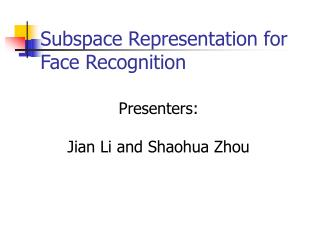# Subspace Representation for Face Recognition - PowerPoint PPT PresentationDownload PresentationSubspace Representation for Face Recognition

Subspace Representation for Face RecognitionDownload Presentation## Subspace Representation for Face Recognition

- - - - - - - - - - - - - - - - - - - - - - - - - - - E N D - - - - - - - - - - - - - - - - - - - - - - - - - - -
##### Presentation Transcript

1. Subspace Representation for Face Recognition Presenters: Jian Li and Shaohua Zhou

2. Overview • 4 different subspace representations • PCA, PPCA, LDA, and ICA • 2 options • Kernel v.s. Non-Kernel • 2 databases with 3 different variations • Pose, Facial expression, and Illumination

3. Subspace representations • Training data X (d,n) • X = [x1, x2, …, xn] • Subspace decomposition matrix W (d,m) • W = [w1, w2, …, wm] • Representation Y (m,n) • Y = W’ * X

4. PCA, PPCA, LDA and ICA • PCA, in an unsupervised manner, minimizes the representation error ||X – Y||. • LDA, in a supervised manner, minimizes the within-class distance while maximizing the between-class distance. • ICA, in an unsupervised manner, maximizes the independence between Y ’s. • Probabilistic PCA, coming late …

5. Kernel or Non-Kernel • Often somewhere reduces to some forms related to dot product • Kernel trick • Replacing dot product by kernel function • Mapping the original data space into a high-dimensional feature space • K(x,y) = <f(x) , f(y)> • Gaussian kernel: exp(- 0.5 |x – y|^2/sigma^2)

6. Gallery, Probe, Pre-processing • Training dataset • Testing dataset • Gallery: Reference images in testing • Probe: Probe images in testing • Pre-processing • Down-sampling • Zero-mean-unit-variance • x = { x - mean(x) } / var(x) • Crop face region only

7. AT&T Database • Pose variation • 40 classes, 10 images/class, 28 by 23 Set1 Set2 (Mirror of Set1)

8. FERET Database • Facial expression and illumination variation • 200 classes, 3 images/class, 24 by 21 Set1 Set2 Set3

9. Probabilistic PCA (PPCA) -- I • PCA only extracts PCs thereby losing probabilistic flavor • PPCA add this by interpreting the reconstruction error as confidence level • y = u + W * x + e • Different choices of e • Factor analysis, • PPCA (Tipping and Bishop ’99) • PCA

10. Probabilistic PCA (PPCA) -- II • Assume e has covariance matrix, pho*I • R = U * D * U’ • W = Um * (Dm – pho*I) ^(1/2) • Pho = mean of the remaining eigenvalues • Implemented algorithm • B. Moghaddam ’01 • W = Um * (Dm) ^(1/2) • - 2log P(y) = sum (Pci^2/Di) + e^2 / pho + const • Construct inter-person space

11. Probabilistic KPCA (PKPCA) • Replace PCA by KPCA in the PPCA algorithm • Estimating e by computing sum of all remaining PC’s.

12. ICA • Independent face • PCA pre-whitening: X1 = U’ * X • Y = W * X1 • Independent facial expression • Y = W * X’

13. Kernel ICA • F. Bach and M. I. Jordan ‘01 • ‘Kernel trick’ is played when measuring independence • Canonical correlation -- independence

14. Experimental Setup • Training • Ranking the gallery based on the distance or probability • CMS curve

15. Distance Metric • SAD, SQD, Correlation (mean removed)

16. Tweaking Gaussian kernel width

17. Eigenfaces & Fisherfaces Eigenfaces Fisherfaces

18. Independent Basis Faces & Facial Features Ind. Faces Ind. Facial Features

19. Performance on pose variation

20. Performance on facial expression variation

21. Performance on illumination variation

22. Comparison of 4 methods

23. Comparison of Kernel/Non-kernel methods

24. Computational load • Training time: • PCA < LDA < PPCA < ICA • KPCA < KLDA < PKPCA << KICA • Testing time: • PCA = LDA = ICA < PPCA • KPCA = KLDA = KICA < PKPCA